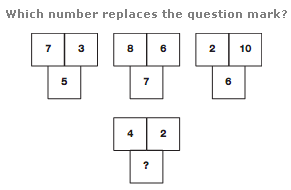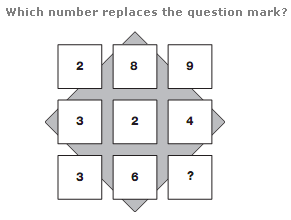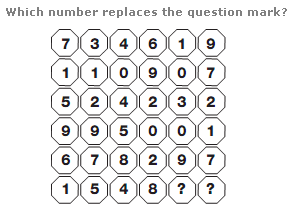# Puzzles - Number puzzles

Exercise : Number puzzles
13.:
3
Explanation
:
In each group of 3 numbers, the lower number equals the average of the top two numbers.

14.:
1
Explanation
:
Reading each row as a 3 digit number, the rows follow the sequence of square numbers, from 17 to 19.

15.